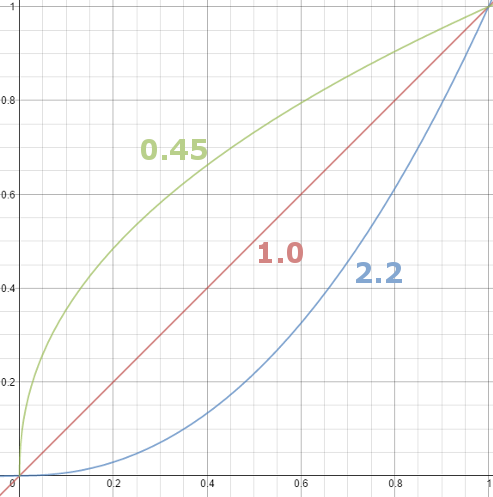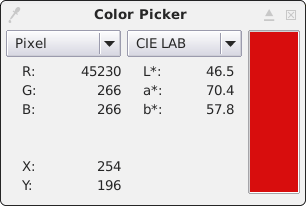#rigacci.org

## Energia

Rigacci.Org usa energia elettrica da fonti rinnovabili, grazie al gruppo di acquisto Merci Dolci.

## Software libero!

doc:appunti:software:color_management

## Definitions

### Color models

A color model is a method to turn colors into numbers.

#### RGB

The RGB model uses three primary addictive colors, it is used by digital cameras and monitors. The three numbers are generally expressed in a range from 0 to the max number of bits used per channel (0-255 for 8 bits, 0-65535 for 16 bits, etc.)

#### CMYK

The CMYK model uses four subtractive colors, it is modeled on how inks absorb colored light. It is used by many printing process.

#### CIEXYZ

Many monochromatic light beams (in the visible 380-780 nm spectrum) were projected one at a time onto a screen. For each beam (wavelength), three values were determined: the intensity of three beams of primary colors (red, green and blue) that need to be mixed to produce the same visual perception of the monochromatic light. The test was done on a sample of people taken as reference.

The X, Y and Z are not the color components. The Y values is the luminance, the Z value represents somewhat the blue component and the X represents the remaining dimension, adjusted to be orthogonal and non-negative.

#### CIELAB

This color model (also called L*,a*,b* or Lab or CIE 1976), uses three numbers representing respectively: the lightness, the position on the red-green and the position on the yellow-blue. The L* values is generally expressed with a number in the range 0-100, the a* and b* values ranges from negative to positive (e.g. -127 to +127 for an 8 bit value), where the zero represents the color-neutral position (where L* = 0 is black and L* max is white).

It is derivated from the CIEXYZ model, with the added benefit that equal differences in numerical values correspond to equal differences in color perception. It is modeled upon human vision and designed to be device-independent.

### Linear vs non-linear color spacesIn a linear color space the numerical intensity values corresponds proportionally to their perceived intensity. This is roughly how light works: if you sum two equal light rays, the resulting perceived intensity is doubled. So the best choice should be to use a linear model of colors. But there are two facts:

• Screens have a non-linear response to signal intensity.
• The human eye can tell the difference between darker shades better than lighter shades, so it is desiderable a greater accuracy for dark intensities at the expense of lighter intensities.

For these reasons, an exponential function (where the exponent is called gamma) is applied to the values of light intensity. Numeric values are stored generally applying a gamma (exponent) of 0.45. This has the advantage that numerical values can be sent to the screen in a more direct way. The gamma function will also give some advantages to the precision of dark shades when numerical values are casted to integers, expecially when only 8 bits are used for each color channel.

To convert the stored numerical values back to light intensity, an exponent of 2.2 must be applied. Notice that a linear color space can be seen as a non-linear space where the exponent gamma is 1.0.

So the sRGB (non linear) color space is widely used; that model is often called perceptual gamma. But if you work with high quality images (16 bits per channel) and if you want to apply filters that work on light and shadow, you should use a linear (RBG) workspace. The GIMP 2.10 program internally uses linear channels data when higher than 8-bit per channel precision is used.

### Color spaces

A color space is some specific implementation of a color model. The extent of a colors space (the range of representable colors) is called gamut. Some color spaces are wider (contains more colors) than others.

#### sRGBsRGB was developed by HP and Microsoft in 1996, it is based on the RGB model. It is a standard for displaying signals (e.g. high-definition television, HDTV) used by LCD, digital cameras, scanners, CRT (using some compensating circuits). Generally used for graphic files, it is considered the default color space in absence of any embedded profile. Generally it uses 8 bits per channel. It uses the D65 as the white point.

It is a non-linear color space. Numerical values and perceived light/color intensity are not linked by a simple multiplier function, but an exponential one is used. The exponent (called gamma) is roughly 2.2; actually it is a variable number ranging from 1.0 to 2.3. In the first part of the graph (for x = 0 - 0.04045) it has a constant value 1.0.

In the graph to the right the red line is the sRGB gamma curve, which is very near the 2.2 gamma curve (dotted black line). The blue line is the variable gamma exponent used.

Defined by Adobe in 1998, it has a gamut wider than sRGB, mainly in the cyan-green zone. It is a non-linear color space with gamma exponent 2.2. It covers the 52.1% of CIE 1931 color space.

#### ProPhoto RGB

Designed as a universal standard for high-bit image editing. Not suitable for 8bit/channels images, can also suffer of out-of-gamut color mapping when converting to RGB or CMYK.

#### CIE 1931 RGB

The CIE 1931 color space were the first defined quantitative links between wavelengths in the visible spectrum, and physiologically perceived colors in human color vision.

#### CIE 1931 XYZ

Derived from the CIE RGB color space.

#### Rec. 709

ITU-R BT.709 standard for the high-definition television (HDTV). It covers the 35.9% of CIE 1931 color space.

#### Rec. 2020

ITU-R Recommendation BT.2020. Defines various aspects of ultra-high-definition television (UHDTV). Can reproduce colors that cannot be shown with the Rec. 709 (HDTV) color. Defines linear RGB: 0.0 to 1.0 with a bit depth of either 10 bits per sample or 12 bits per sample. It covers the 75.8% of CIE 1931 color space.

## The GIMP

The GIMP version 2.10 is required for color management and to handle TIFF images with 16 bit per color channel.To view CIE LAB values in Color Picker: select the Color Picker tool, then keep the shift key pressed while clicking over the image.

## Other Interesting Pages